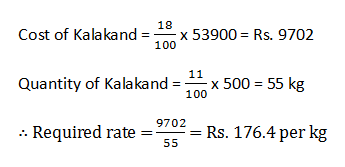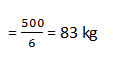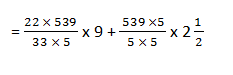# SBI PO Data Interpretation Series - Set 5

Directions (1-5): Study the pie- charts carefully and answer the following questions:
At a sweet corner 500 kg of different types of sweets are sold per day, which is equivalent to Rs. 53900.Quantity in terms of WeightQuantity in terms of Price

Ques 1. At what rate does the shopkeeper sell Kalakand?
1. Rs. 174.6 per kg
2. Cannot be determined
3. Other than the given options
4. Rs. 178.6 per kg
5. Rs. 176.4 per kg

Ques 2. What is the approximate average of different types of sweets sold per day?
1. Other than the given options
2. 83 kg
3. 79 kg
4. 80 kg
5. 84 kg

Ques 3. Which of the following sweets is the costliest?
1. Pinni
2. Burfi
3. Kalakand
5. Milkcake

Ques 4. What money should be paid by Preeti for 9 kg of Gulab Jamun and two and a half kg of Milacake?
1. Rs. 906.8
2. Rs. 916.3
3. Other than the given options
4. Rs. 816.3
5. Rs. 926.5

Ques 5. If the shopkeeper sells 11.5 kg more Pinni what is the total amount fetched?
1. Rs. 55355.3
2. Rs. 55455.3
3. Rs. 57855.3
4. Other than the given options
5. Rs. 56355.3

1. 5;2. 2;
Required average3. 3;
Cost of sweets per kg (in Rs.)Hence, the costliest sweet is Kalakand.

4. 2;
Required amount= 646.8 + 269.5 = Rs. 916.3

5. 1;
Total amount fetched= Rs. 55355.3